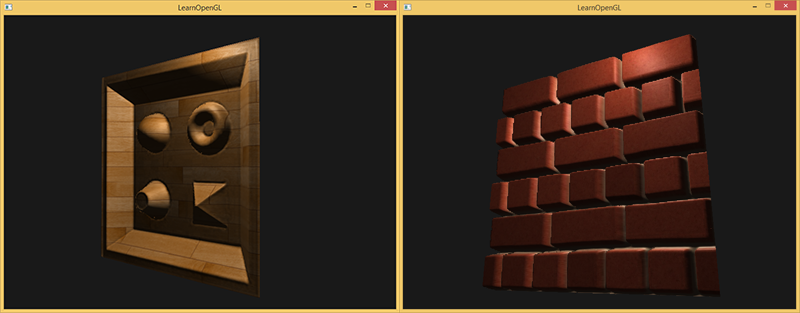# 视差贴图

Note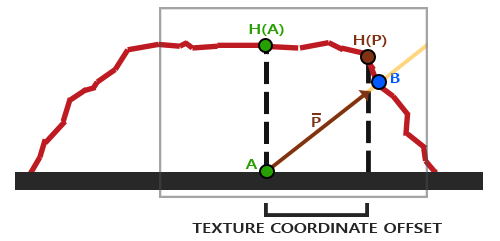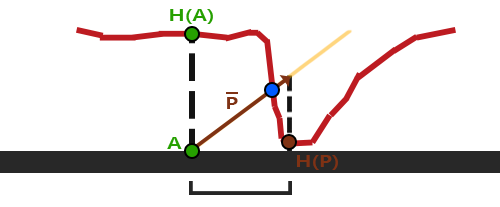## 视差贴图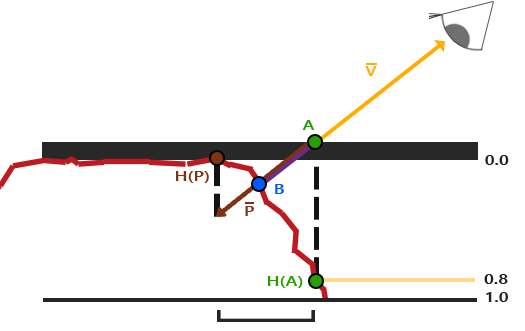#version 330 core
layout (location = 0) in vec3 position;
layout (location = 1) in vec3 normal;
layout (location = 2) in vec2 texCoords;
layout (location = 3) in vec3 tangent;
layout (location = 4) in vec3 bitangent;

out VS_OUT {
vec3 FragPos;
vec2 TexCoords;
vec3 TangentLightPos;
vec3 TangentViewPos;
vec3 TangentFragPos;
} vs_out;

uniform mat4 projection;
uniform mat4 view;
uniform mat4 model;

uniform vec3 lightPos;
uniform vec3 viewPos;

void main()
{
gl_Position      = projection * view * model * vec4(position, 1.0f);
vs_out.FragPos   = vec3(model * vec4(position, 1.0));
vs_out.TexCoords = texCoords;

vec3 T   = normalize(mat3(model) * tangent);
vec3 B   = normalize(mat3(model) * bitangent);
vec3 N   = normalize(mat3(model) * normal);
mat3 TBN = transpose(mat3(T, B, N));

vs_out.TangentLightPos = TBN * lightPos;
vs_out.TangentViewPos  = TBN * viewPos;
vs_out.TangentFragPos  = TBN * vs_out.FragPos;
}


#version 330 core
out vec4 FragColor;

in VS_OUT {
vec3 FragPos;
vec2 TexCoords;
vec3 TangentLightPos;
vec3 TangentViewPos;
vec3 TangentFragPos;
} fs_in;

uniform sampler2D diffuseMap;
uniform sampler2D normalMap;
uniform sampler2D depthMap;

uniform float height_scale;

vec2 ParallaxMapping(vec2 texCoords, vec3 viewDir);

void main()
{
// Offset texture coordinates with Parallax Mapping
vec3 viewDir   = normalize(fs_in.TangentViewPos - fs_in.TangentFragPos);
vec2 texCoords = ParallaxMapping(fs_in.TexCoords,  viewDir);

// then sample textures with new texture coords
vec3 diffuse = texture(diffuseMap, texCoords);
vec3 normal  = texture(normalMap, texCoords);
normal = normalize(normal * 2.0 - 1.0);
// proceed with lighting code
[...]
}


vec2 ParallaxMapping(vec2 texCoords, vec3 viewDir)
{
float height =  texture(depthMap, texCoords).r;
vec2 p = viewDir.xy / viewDir.z * (height * height_scale);
return texCoords - p;
}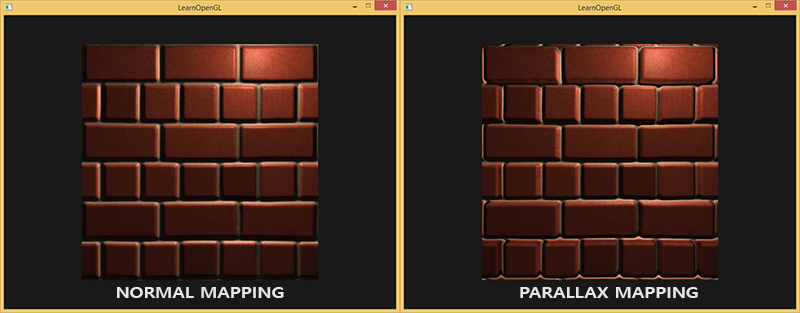texCoords = ParallaxMapping(fs_in.TexCoords,  viewDir);
if(texCoords.x > 1.0 || texCoords.y > 1.0 || texCoords.x < 0.0 || texCoords.y < 0.0)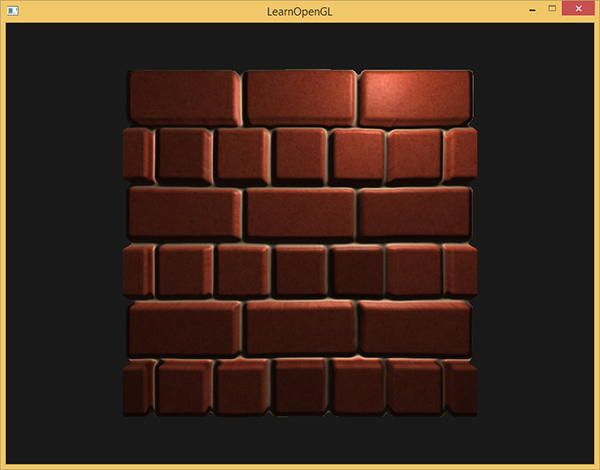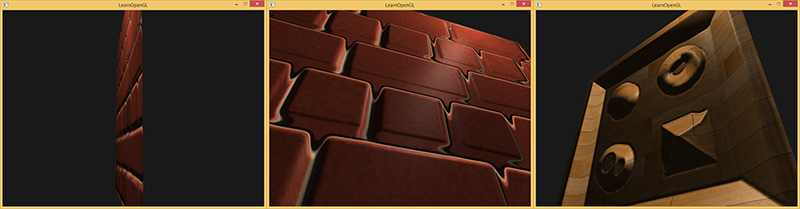## 陡峭视差映射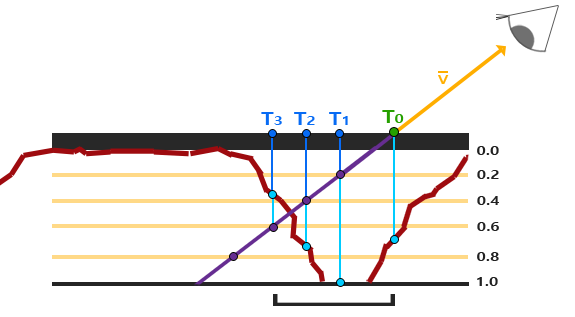vec2 ParallaxMapping(vec2 texCoords, vec3 viewDir)
{
// number of depth layers
const float numLayers = 10;
// calculate the size of each layer
float layerDepth = 1.0 / numLayers;
// depth of current layer
float currentLayerDepth = 0.0;
// the amount to shift the texture coordinates per layer (from vector P)
vec2 P = viewDir.xy * height_scale;
float deltaTexCoords = P / numLayers;

[...]
}


// get initial values
vec2  currentTexCoords     = texCoords;
float currentDepthMapValue = texture(depthMap, currentTexCoords).r;

while(currentLayerDepth < currentDepthMapValue)
{
// shift texture coordinates along direction of P
currentTexCoords -= deltaTexCoords;
// get depthmap value at current texture coordinates
currentDepthMapValue = texture(depthMap, currentTexCoords).r;
// get depth of next layer
currentLayerDepth += layerDepth;
}

return texCoords - currentTexCoords;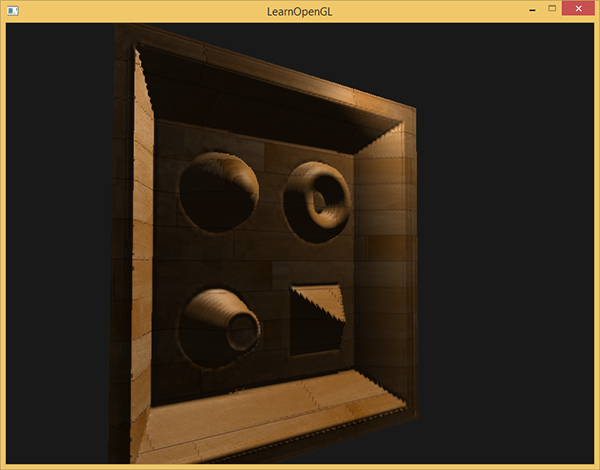const float minLayers = 8;
const float maxLayers = 32;
float numLayers = mix(maxLayers, minLayers, abs(dot(vec3(0.0, 0.0, 1.0), viewDir)));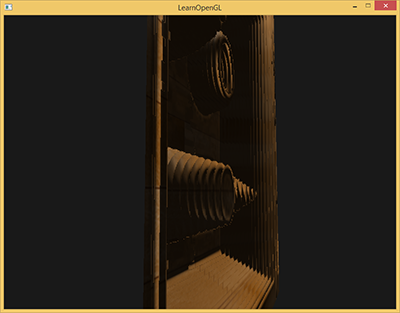## 视差遮蔽映射

[...] // steep parallax mapping code here

// get texture coordinates before collision (reverse operations)
vec2 prevTexCoords = currentTexCoords + deltaTexCoords;

// get depth after and before collision for linear interpolation
float afterDepth  = currentDepthMapValue - currentLayerDepth;
float beforeDepth = texture(depthMap, prevTexCoords).r - currentLayerDepth + layerDepth;

// interpolation of texture coordinates
float weight = afterDepth / (afterDepth - beforeDepth);
vec2 finalTexCoords = prevTexCoords * weight + currentTexCoords * (1.0 - weight);

return finalTexCoords;